# Numerical analysis

(diff) ← Older revision | Latest revision (diff) | Newer revision → (diff)

The branch of mathematics concerned with finding accurate approximations to the solutions of problems whose exact solution is either impossible or infeasible to determine. In addition to the approximate solution, a realistic bound is needed for the error associated with the approximate solution. Typically, a mathematical model for a particular problem, generally consisting of mathematical equations with constraint conditions, is constructed by specialists in the area concerned with the problem. Numerical analysis is concerned with devising methods for approximating the solution to the model, and analyzing the results for stability, speed of implementation, and appropriateness to the situation.

## Error considerations.

Some major difficulties confront the numerical analyst when attempting to determine an effective approximation technique. The first concerns the nature of calculations in the real world. As a mathematician and scientist, one assumes that there is an infinite number of real numbers, and that each real number has an infinite number of neighbours arbitrarily close to it. Idealized computation takes into consideration all these real numbers, but practical calculation can consider only a small subset. For example, in idealized calculation one can have. In a calculator or computer, the irrational number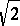is given an approximate representation using a fixed finite number of digits, and the squaring operation is performed on this finite-digit approximation. The result of the calculation within the computer is close to, but not exactly the same as, the real number. Additional operations using inexact numbers of this type can lead to significant errors in approximation. The discipline of numerical analysis involves the design of techniques that take these and other error-producing situations into account when approximating the solution to a problem.

The difference between the true value of a real number and its finite-digit approximation within a computer is called the round-off error associated with the number. This round-off error depends not only on the real number but also on the architecture of the computer on which it is being represented and, very likely, the programming system that is used for the calculations. Approximation techniques must consider the effect of this round-off error and its propagation when the finite-digit approximations are used in subsequent calculations. In general, the total round-off error grows as a function of the number of computations, so efficient approximation techniques attempt to control the number of calculations for both computational and accuracy effectiveness.

Certain approximation procedures are more effected by round-off error difficulties than others. Methods in which small errors in representation are likely to produce large errors in the final approximation are said to be unstable. Derivative approximation techniques are generally unstable; for example, the error associated with finite-digit arithmetic can completely dominate the calculations in some derivative approximations. Techniques in which the round-off error plays little role in the accuracy of the computation are called stable. Approximating definite integrals (cf. also Definite integral) is generally stable, since round-off is likely to have little effect on the final approximation, regardless of the number of calculations. The effect of the round-off error on problems involving matrix operations is likely to depend on the specific matrices that are used in the computations. In some cases the round-off error will be negligible, in others it will grow dramatically and dominate the approximation. Knowing how to construct methods of approximation that will minimize the effect of round-off error on the final results is one of the basic tools of the numerical analyst.

An associated error difficulty is the representation of the problem itself. It is seldom the case that the mathematical model precisely represents the physical problem that the model's constructor wishes to study. A desirable feature for an approximation method, then, is that the solution to a realistic approximation to a problem produces a good approximation to not only the mathematical model, but the physical problem as well. Small changes in a problem are called perturbations of the problem. Perturbations might occur because of small errors in the statement of the problem or they might be designed into the approximation problem to allow a solution to be more easily determined. In a problem with many variables, such as a weather or economic prediction model, an approximate solution to a problem might only be feasible if it is assumed that certain of the variables can be ignored, and that all the other variables in the problem effect the final solution only in a linear manner. Even though this is not likely to be the true situation, the model based on these assumptions might lead to useful predictions if the model is stable with respect to the perturbations that these assumptions force on the problem.

In addition to round-off error considerations associated with the solution to a problem, there is the fact that many true solutions involve an infinite number of calculations, for example, when the true solution to a problem is known to be associated with an infinite series, such as a Taylor series. A standard procedure in approximation methods is to use only a finite number of terms of the series to represent the entire series. The error associated with the truncation of the infinite series to a finite series is called truncation error. How this truncation error effects the accuracy of the final approximation result depends on the stability of the technique to this type of error.

## Solution of linear equations.

Determining the solution,, to a single linear equation of the formfor a given value ofis simply a matter of algebraic manipulation, provided that. In a similar manner, there are straight-forward, or direct, methods (cf. also Direct method) for determining the solution for systems of linear equations of the form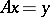, whereis an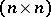-matrix andandare-dimensional vectors. The most common direct method is Gaussian elimination (cf. Elimination theory), which will produce the exact solution to the linear system using on the order ofarithmetic operations. Such a solution would not call for numerical analysis, except that the calculations generally involve finite-digit approximations of the numbers in the matrixand the given vector. In some cases the accumulation of round-off errors cannot be avoided, to the extent that the results may be meaningless, but often the calculations can be arranged to minimize the propagation of this round-off error.

Some large linear systems have the property that the associated matrix has only a relatively small number of non-zero entries and these are often concentrated along the main diagonal of the matrix. Matrices with this feature are called sparse (cf. also Sparse matrix). It is often appropriate to approximate the solution to a sparse linear system by assuming an initial approximation to the solution and using a recursive procedure to successively generate improvements. The classical techniques of Jacobi (cf. Jacobi method) and Gauss–Seidel (cf. also Gauss method; Seidel method) solve theth equation in the system for the variable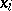and use known previous approximations to determine, in order, new approximations for. More sophisticated techniques improve on the new approximations by appropriately weighting the previous approximations or by generating approximations on subspaces. The effective approximation to the solution of systems of linear equations is particularly important in numerical analysis because the solutions to many other approximation problems either are approximated by a linear system or have a subportion that requires the solution of a linear system.

## Solution of non-linear equations.

One of the earliest and most basic problems of numerical analysis concerns the approximation of solutions to a single non-linear equation. Even for the simplest of equations, those involving polynomials (cf. also Polynomial), there is no procedure that will produce exact results in most situations. Methods for approximating the solutions to equations have been used almost from the beginning of recorded mathematical time, and most are iterative in nature. They begin with some approximate solutions to the equation, and improve on the approximations using a recursive procedure. Often, this recursive procedure uses a Taylor polynomial to replace the function involved in the equation; the most popular elementary technique being the Newton method, which in various forms has been used since Antiquity. This technique for approximating the solution to an equation of the form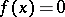requires one initial approximation to the solution and uses the intersection of the-axis with the tangent line to the graph ofto give an improved approximation. This is often a very effective technique, with the approximations doubling their accuracy with each iteration. It is not, however, effective in all circumstances, and there are many other choices that may be more appropriate for a particular problem.

Methods for determining the simultaneous solution to a number of equations in a number of unknowns generally follow the pattern of the single-equation methods. The functions determining the equations are expressed as a vector function with vector variables, and although some modification of the notion of the various derivatives of these functions is required, the procedure is similar. As the number of equations and variables increases, the difficulty with obtaining a sufficiently accurate starting approximation for the recursive methods can become overwhelming. In this case it might be more effective to use techniques such as those that involve following a continuous path from the solution of a known problem to the solution of the problem in question.

## Approximation of data and functions.

There are many situations that call for fitting a common function to a collection of data. Determining a function that agrees precisely with the data, at least to within round-off tolerances, is called interpolation. In the case of an arbitrary collection of data, polynomial interpolation is the most likely candidate for mainly pragmatic reasons. A polynomial approximation to a collection of data is easy to determine in various ways, and polynomials have easily computed derivatives and integrals that might be useful if the derivative or integral of the function underlying the data is needed. However, a polynomial of degreeis generally required to satisfy a set ofconditions, and polynomials of even moderately high degree are likely to have a high degree of variation except where explicitly constrained. This means that in order to obtain stable approximating polynomials either the number of specified conditions must be kept small, which may not be a reasonable restriction, or, more likely, the conditions are only required to be satisfied in an approximate way.

Spline function interpolations (cf. also Spline interpolation) use piecewise polynomials of fairly low degree to overcome some of the polynomial variation weaknesses. They are constructed so that a fixed-degree polynomial is used as an approximation between consecutive data points, with the specific polynomial of that degree changing as the pair of data points changes. For example, by fitting cubic polynomials between pairs of consecutive data points there is sufficient flexibility to ensure the approximating function has a continuous second-order derivative. By using higher-degree polynomials between the points, higher differentiability conditions can be imposed. Modifications of the spline procedure can be made to guarantee that the approximating piecewise polynomial will satisfy user-specified conditions concerning the direction that the curve will assume at the data points. This is particularly important when the resulting curve needs to satisfy aesthetic conditions needed in computer graphics routines.

Interpolation is not the only possibility for data approximation, and may not be the technique of choice, even when the approximating function is a polynomial. The method of least squares (cf. Least squares, method of) is a classic method of controlling the variability on an approximating polynomial. This technique determines the polynomial of a fixed degree that "best" approximates a given set of data. Although the least-squares polynomial that is constructed may not agree precisely with any of the data, it has the property that the sum of the squares of the differences between the approximate ordinates and the ordinates associated with the data is as small as possible. Other criteria are used to construct the "best" polynomial that fits a given collection of data, but the least-squares procedure is the most popular.

The problem of approximating a known function with a simpler function follows a pattern similar to that of fitting a function to a collection of data. There are many reasons why such an approximation might be required. For example, suppose one needs to know the value of the definite integral of a given function. In only the most elementary situations will it be possible to evaluate this integral directly, so some approximation technique is required. The basic underlying technique involves fitting a polynomial to a collection of data generated by the function and using the definite integral of this polynomial to approximate the integral of the function.

It might also be the case that one needs values of a particular function to use in some approximating procedure and evaluating the function is computationally expensive or time consuming or particularly inconvenient; for example, when the approximation is needed in a computer program and the definition of the function lies outside the program. In this case a sufficiently accurate approximation to the function may not affect the error in the final approximation and may be much easier or cheaper to determine.

When approximating a known function with a simpler function, there is flexibility in the choice of data since the data in this case is obtained by evaluating the function at specified points. In some cases one may wish the data to be equally-spaced; in others it can be chosen so that more data is available at points of special interest, perhaps when there appears to be a rapid change in the values of the function or its derivatives.

Periodic data and functions are more appropriately approximated using the basic periodic functions, the sine and cosine. These techniques are called Fourier approximation methods and are very commonly used in applications such as signal processing (cf. also Fourier series). Large amounts of data are sampled, generally at equally-spaced time intervals, with the objective of quickly determining a periodic function that approximates the data. Because of the large amount of calculation involved, the data are sampled in ways that permit this calculation to be done extremely efficiently, using techniques know as fast Fourier transforms.

All of these techniques extend to functions of multiple variables, although the amount of computation increases with the number of variables. When this increase is a polynomial function of the number of variables, there is an inherent stability in the procedure, but computation that increases exponentially with the number of variables produces instability.

## Approximation of integrals and derivatives.

Integration is extremely important in applications, but very rarely is it possible to obtain the exact value of a definite integral since this requires an anti-derivative of the function. When one cannot find the anti-derivative, an approximation method is needed. The definition of the definite integral involves the limit of sums involving evaluations of the function (cf. also Riemann integral; Integral sum), which provides a natural way for approximating the integral. It is a very inefficient procedure, however, and much better approximation methods are obtained by using an interpolating function to approximate the function and then integrating the interpolating function to produce the approximate value for the integral. The interpolating functions that are generally used are polynomials or piecewise polynomials. Numerical integration procedures are generally stable and can be designed to incorporate accuracy checks to modify the technique if it is suspected that the approximation is not sufficiently accurate.

Since the definition of the derivative involves the limit of the ratio of the change in the values of a function with respect to the change in its variable, the basic derivative approximation techniques use a difference quotient to approximate the derivative of a given function. However, numerical differentiation is generally unstable with respect to round-off error, and, unless care is used, the resulting approximations may be meaningless.

## Differential equations.

Approximating the solution to ordinary and partial differential equations is one of the most common problems of numerical analysis (cf. also Differential equations, ordinary, approximate methods of solution of; Differential equation, partial, variational methods; Elliptic partial differential equation, numerical methods; Hyperbolic partial differential equation, numerical methods; Parabolic partial differential equation, numerical methods). There is a connection between determining the solution of a differential equation and the evaluation of a definite integral, and since relatively few definite integrals can be determined exactly, the same is true for differential equations. The basic techniques for approximating the solution,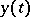, for a single differential equation with a specified initial value (cf. also Approximation of a differential boundary value problem by difference boundary value problems), of the formuse the differential equation to determining the slope ofatto predict the value of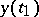for some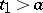. This approximation is used in the same manner to predict the value offor some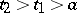, and so on. Interpolation can be used to determine approximations toat intermediate values of.

One-step approximation methods use only the most recently calculated approximation at each step, whereas multi-step methods use a number of previous approximations to compute each new approximation. The most common one-step methods are the Runge–Kutta techniques (cf. Runge–Kutta method), and the Adams' predictor-corrector methods (cf. Adams method) are commonly used multi-step techniques. More sophisticated methods incorporate procedures to approximate the amount of error at each step, and provide automatic adjustments that keep the overall error within a specified bound.

Systems of differential equations are solved using modifications of the single-equation techniques. Since the methods generally proceed stepwise from one approximation to the next, the calculations need to be arranged so that all the previous approximations are available when a new approximation is being calculated. Approximations to the solutions of higher-order differential equation are obtained by converting the higher-order equation into a system of differential equations.

There are a number of approximation techniques available for solving boundary value problems associated with ordinary differential equations (cf. also Boundary value problem, ordinary differential equations). The shooting methods (cf. Shooting method) convert the boundary value problem (cf., e.g., Boundary value problem, ordinary differential equations) to a collection of initial-value problems and then use some of the methods discussed previously. Finite-difference methods (cf. Finite-difference calculus) approximate the derivatives in the boundary value problem with approximations that permit the problem to be changed into a linear system. Variational techniques consider an equivalent problem that involves minimizing the value of an integral. Although this minimization problem cannot be solved exactly, the solution to the problem can be approximated by determining the minimal value over certain classes of basis functions.

Approximate solutions to partial differential equations can be found by applying finite-difference and variational techniques similar to those used for ordinary boundary value problems. For the ordinary boundary value problems, the boundary consists of only two points, the end points of an interval. The boundary of a partial differential equation, on the other hand, will likely be a collection of curves in the plane or surfaces in space. When the boundary is difficult to express the finite-difference techniques suffer, but the variational, or finite-element, techniques can accommodate a wide range of boundaries. Cf. also Boundary value problem, partial differential equations; Boundary value problem, numerical methods for partial differential equations.

There is a wide variety in the type of problems numerical analysts attempt to solve, and only some of the more important topics have been mentioned. Because of the variety of problems and techniques, there is a vast body of literature on the subject. Those listed below can be consulted for a more complete discussion.

How to Cite This Entry:
Numerical analysis. Encyclopedia of Mathematics. URL: http://encyclopediaofmath.org/index.php?title=Numerical_analysis&oldid=12739
This article was adapted from an original article by Doug Faires (originator), which appeared in Encyclopedia of Mathematics - ISBN 1402006098. See original article